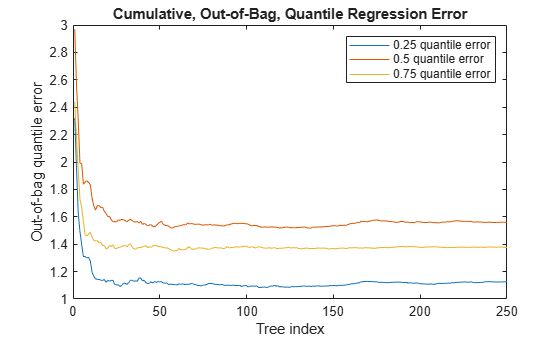# oobQuantileError

Class: TreeBagger

Out-of-bag quantile loss of bag of regression trees

## Syntax

``err = oobQuantileError(Mdl)``
``err = oobQuantileError(Mdl,Name,Value)``

## Description

example

````err = oobQuantileError(Mdl)` returns half of the out-of-bag mean absolute deviation (MAD) from comparing the true responses in `Mdl.Y` to the predicted, out-of-bag medians at `Mdl.X`, the predictor data, and using the bag of regression trees `Mdl`. `Mdl` must be a `TreeBagger` model object.```

example

````err = oobQuantileError(Mdl,Name,Value)` uses additional options specified by one or more `Name,Value` pair arguments. For example, specify quantile probabilities, the error type, or which trees to include in the quantile-regression-error estimation.```

## Input Arguments

expand all

Bag of regression trees, specified as a `TreeBagger` model object created by `TreeBagger`.

• The value of `Mdl.Method` must be `regression`.

• When you train `Mdl` using `TreeBagger`, you must specify the name-value pair `'OOBPrediction','on'`. Consequently, `TreeBagger` saves required out-of-bag observation index matrix in `Mdl.OOBIndices`.

### Name-Value Arguments

Specify optional pairs of arguments as `Name1=Value1,...,NameN=ValueN`, where `Name` is the argument name and `Value` is the corresponding value. Name-value arguments must appear after other arguments, but the order of the pairs does not matter.

Before R2021a, use commas to separate each name and value, and enclose `Name` in quotes.

Ensemble error type, specified as the comma-separated pair consisting of `'Mode'` and a value in this table. Suppose `tau` is the value of `Quantile`.

ValueDescription
`'cumulative'`

`err` is a `Mdl.NumTrees`-by-`numel(tau)` numeric matrix of cumulative quantile regression errors. `err(j,k)` is the `tau(k)` quantile regression error using the learners in `Mdl.Trees(1:j)` only.

`'ensemble'`

`err` is a 1-by-`numel(tau)` numeric vector of cumulative quantile regression errors for the entire ensemble. `err(k)` is the `tau(k)` ensemble quantile regression error.

`'individual'`

`err` is a `Mdl.NumTrees`-by-`numel(tau)` numeric matrix of quantile regression errors from individual learners. `err(j,k)` is the `tau(k)` quantile regression error using the learner in `Mdl.Trees(j)` only.

For `'cumulative'` and `'individual'`, if you choose to include fewer trees in quantile estimation using `Trees`, then this action affects the number of rows in `err` and corresponding row indices.

Example: `'Mode','cumulative'`

Quantile probability, specified as the comma-separated pair consisting of `'Quantile'` and a numeric vector containing values in the interval [0,1]. For each observation (row) in `Mdl.X`, `oobQuantileError` estimates corresponding quantiles for all probabilities in `Quantile`.

Example: `'Quantile',[0 0.25 0.5 0.75 1]`

Data Types: `single` | `double`

Indices of trees to use in response estimation, specified as the comma-separated pair consisting of `'Trees'` and `'all'` or a numeric vector of positive integers. Indices correspond to the cells of `Mdl.Trees`; each cell therein contains a tree in the ensemble. The maximum value of `Trees` must be less than or equal to the number of trees in the ensemble (`Mdl.NumTrees`).

For `'all'`, `oobQuantileError` uses all trees in the ensemble (that is, the indices `1:Mdl.NumTrees`).

Values other than the default can affect the number of rows in `err`.

Example: `'Trees',[1 10 Mdl.NumTrees]`

Data Types: `char` | `string` | `single` | `double`

Weights to attribute to responses from individual trees, specified as the comma-separated pair consisting of `'TreeWeights'` and a numeric vector of `numel(trees)` nonnegative values. `trees` is the value of `Trees`.

If you specify `'Mode','individual'`, then `oobQuantileError` ignores `TreeWeights`.

Data Types: `single` | `double`

## Output Arguments

expand all

Half of the out-of-bag quantile regression error, returned as a numeric scalar or `T`-by-`numel(tau)` matrix. `tau` is the value of `Quantile`.

`T` depends on the values of `Mode`, `Trees`, and `Quantile`. Suppose that you specify `'Quantile',tau` and `'Trees',trees`.

• For `'Mode','cumulative'`, `err` is a `numel(trees)`-by-`numel(tau)` numeric matrix. `err(j,k)` is the `tau(k)` cumulative, out-of-bag quantile regression error using the learners in `Mdl.Trees(trees(1:j))`.

• For `'Mode','ensemble'`, `err` is a `1`-by-`numel(tau)` numeric vector. `err(k)` is the `tau(k)` cumulative, out-of-bag quantile regression error using the learners in `Mdl.Trees(trees)`.

• For `'Mode','individual'`, `err` is a `numel(trees)`-by-`numel(tau)` numeric matrix. `err(j,k)` is the `tau(k)` out-of-bag quantile regression error using the learner in `Mdl.Trees(trees(j))`.

## Examples

expand all

Load the `carsmall` data set. Consider a model that predicts the fuel economy of a car given its engine displacement, weight, and number of cylinders. Consider `Cylinders` a categorical variable.

```load carsmall Cylinders = categorical(Cylinders); X = table(Displacement,Weight,Cylinders,MPG);```

Train an ensemble of bagged regression trees using the entire data set. Specify 100 weak learners and save the out-of-bag indices.

```rng(1); % For reproducibility Mdl = TreeBagger(100,X,'MPG','Method','regression','OOBPrediction','on');```

`Mdl` is a `TreeBagger` ensemble.

Perform quantile regression, and out-of-bag estimate the MAD of the entire ensemble using the predicted conditional medians.

`oobErr = oobQuantileError(Mdl)`
```oobErr = 1.5349 ```

`oobErr` is an unbiased estimate of the quantile regression error for the entire ensemble.

Load the `carsmall` data set. Consider a model that predicts the fuel economy of a car given its engine displacement, weight, and number of cylinders.

```load carsmall X = table(Displacement,Weight,Cylinders,MPG);```

Train an ensemble of bagged regression trees using the entire data set. Specify 250 weak learners and save the out-of-bag indices.

```rng('default'); % For reproducibility Mdl = TreeBagger(250,X,'MPG','Method','regression',... 'OOBPrediction','on');```

Estimate the cumulative; out-of-bag; 0.25, 0.5, and 0.75 quantile regression errors.

`err = oobQuantileError(Mdl,'Quantile',[0.25 0.5 0.75],'Mode','cumulative');`

`err` is an 250-by-3 matrix of cumulative, out-of-bag, quantile regression errors. Columns correspond to quantile probabilities and rows correspond to trees in the ensemble. The errors are cumulative, so they incorporate aggregated predictions from previous trees.

Plot the cumulative, out-of-bag, quantile errors on the same plot.

```figure; plot(err); legend('0.25 quantile error','0.5 quantile error','0.75 quantile error'); ylabel('Out-of-bag quantile error'); xlabel('Tree index'); title('Cumulative, Out-of-Bag, Quantile Regression Error')```All quantile error curves appear to level off after training about 50 trees. So, training 50 trees appears to be sufficient to achieve minimal quantile error for the three quantile probabilities.

expand all

## Tips

The out-of-bag ensemble error estimator is unbiased for the true ensemble error. So, to tune parameters of a random forest, estimate the out-of-bag ensemble error instead of implementing cross-validation.

## References

 Breiman, L. "Random Forests." Machine Learning 45, pp. 5–32, 2001.

 Meinshausen, N. “Quantile Regression Forests.” Journal of Machine Learning Research, Vol. 7, 2006, pp. 983–999.

## Version History

Introduced in R2016b